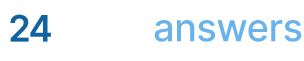# Programming (Dynamic, Linear, Non-linear, etc.)

## Homework Help & Tutoring

We offer an array of different online Programming (Dynamic, Linear, Non-linear, etc.) tutors, all of whom are advanced in their fields and highly qualified to instruct you.Send your subject help request Submit your homework problem, or a general tutoring request.
Get quotes from qualified tutors Receive a response from one of our tutors as soon as possible, sometimes within minutes!
Programming (Dynamic, Linear, Non-linear, etc.) Tutors Available Now
23 tutors available
Programming (Dynamic, Linear, Non-linear, etc.) Homework Library
23 total solutions
See what our students are saying
Can you help me with my homework in less than 24 hours?
Can you help me with my exam/quiz/test?
How much will it cost?
What kind of payments do you accept?

# Programming (Dynamic, Linear, Non-linear, etc.)

The number of problems in math and science to which computer programming methods can be used to solve is virtually unlimited. For such use, many different types of programming algorithms have been developed, each one being useful for specific types of problems. Here are just a few examples of the many types of algorithms available to researchers:

• Approximation algorithms
• Arbitrary precision algorithms
• Bioinformatics algorithms
• Calendar algorithms
• Checksum algorithms
• Classification algorithms
• Combinatorial algorithms
• Compression algorithms
• Concurrent algorithms
• Cryptographic algorithms
• Data clustering algorithms
• Data mining algorithms
• Database algorithms
• Distributed algorithms
• Error detection and correction algorithms
• Evolutionary algorithms
• External memory algorithms
• Genetic algorithms
• Geometric algorithms
• Computer graphics algorithms
• Heuristic algorithms
• Machine learning algorithms
• Memory management algorithms
• Networking algorithms
• Online algorithms
• Optimization algorithms
• Pattern matching algorithms
• Quantum algorithms
• Root-finding algorithms
• Routing algorithms
• Scheduling algorithms
• Search algorithms
• Selection algorithms
• Sorting algorithms
• Statistical algorithms
• Algorithms on strings

A good place to visit periodically to stay up to date with the world of programming algorithms is the Journal of Algorithms.

## College Programming (Dynamic, Linear, Non-linear, etc.) Homework Help

Since we have tutors in all Programming (Dynamic, Linear, Non-linear, etc.) related topics, we can provide a range of different services. Our online Programming (Dynamic, Linear, Non-linear, etc.) tutors will:

• Provide specific insight for homework assignments.
• Review broad conceptual ideas and chapters.
• Simplify complex topics into digestible pieces of information.
• Answer any Programming (Dynamic, Linear, Non-linear, etc.) related questions.
• Tailor instruction to fit your style of learning.

With these capabilities, our college Programming (Dynamic, Linear, Non-linear, etc.) tutors will give you the tools you need to gain a comprehensive knowledge of Programming (Dynamic, Linear, Non-linear, etc.) you can use in future courses.

## 24HourAnswers Online Programming (Dynamic, Linear, Non-linear, etc.) Tutors

Our tutors are just as dedicated to your success in class as you are, so they are available around the clock to assist you with questions, homework, exam preparation and any Programming (Dynamic, Linear, Non-linear, etc.) related assignments you need extra help completing.

In addition to gaining access to highly qualified tutors, you'll also strengthen your confidence level in the classroom when you work with us. This newfound confidence will allow you to apply your Programming (Dynamic, Linear, Non-linear, etc.) knowledge in future courses and keep your education progressing smoothly.

Because our college Programming (Dynamic, Linear, Non-linear, etc.) tutors are fully remote, seeking their help is easy. Rather than spend valuable time trying to find a local Programming (Dynamic, Linear, Non-linear, etc.) tutor you can trust, just call on our tutors whenever you need them without any conflicting schedules getting in the way.

Start Working With Our College Programming (Dynamic, Linear, Non-linear, etc.) Tutors
To fulfill our tutoring mission of online education, our college homework help and online tutoring centers are standing by 24/7, ready to assist college students who need homework help with all aspects of Programming (Dynamic, Linear, Non-linear, etc.).24houranswers.com Parker Paradigms, Inc 5 Penn PLaza, 23rd Floor New York, NY 10001
SUBJECTS COVERED